§ 4 Number Theoretical Functions

A function f ( n ) that has a definite value for any positive integer n is called a number-theoretic function .

[ Integral function and complete integral function ]   If ( m, n ) = 1, there is f ( mn ) = f ( m ) f ( n ), then the number -theoretic function f ( n ) is called an integral function . If for any Positive integers m and n both have f ( mn ) = f ( m ) f ( n ), then f ( n ) is called a completely integral function .

The integral function has the following properties :

1 ° If f ( n ) is a non-zero integral function , then f (1)=1 .

2 ° If both g ( n ) and h ( n ) are integral functions , then g ( n ) h ( n ) are still integral functions . And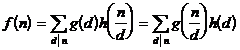is also an integral function , where å is the sum over all the different factors d of n .

3 ° If ​​g ( n ) is a non-zero integral function , and , then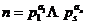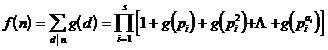is also an integral function .

4 ° If f ( n ) is an integral function , then

f ([ m,n ]) f (( m,n ))= f ( m ) f ( n )

where ( m, n ) is the greatest common factor of m and n , and [ m, n ] is the least common multiple of m and n .

[ Mobius function ]   function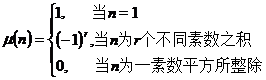called the Mobius function .

The Mobius function has the following properties :

1 °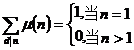2 ° μ ( n ) is an integral function , but not a completely integral function .

3 ° Let , if f ( n ) is an integral function , then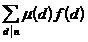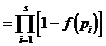is also an integral function . For example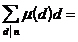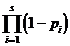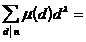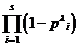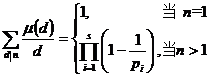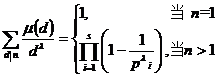[ Eulerian function ]   Let n be a natural number , and ( n ) be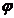the number of positive integers not exceeding n and co -prime to n , called Euler function .

The Euler function has the following properties :

1 ° ( n ) is an integral function , but not a fully integral function .2 ° If , then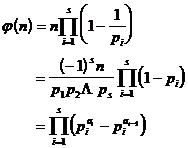In particular , when p is a prime number ,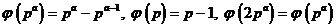3 °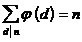4 °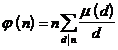[ Divisor function ] The number of all factors of the   natural number n is called the divisor function , denoted as d ( n ). The divisor function has the following properties :

1 ° d ( n ) is an integral function , but it is not a completely integral function . For any natural numbers m, n , there is often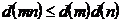2 ° If , then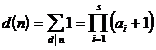[ Von Mangoth function ]   function

L ( n )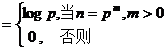is called the von Mangoth function . L ( n ) nonintegral function .

[ Mobius inversion formula and Mobius transformation ]

Once the 1 ° inversion formula is set , let h ( k ) be a non-zero complete integral function . If for all suitable h there is always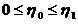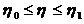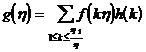Then for the above h also often have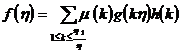The opposite is true .

Let H ( k ) be a non - zero completely integral function . If for all suitable x we ​​have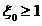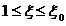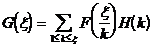Then for the above x also often have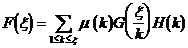The opposite is true .

3 ° Inversion formula 3 is set as , and h ( k ) is set as a non-zero completely integral function . If for all constant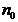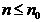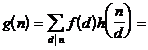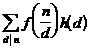Then for the above n also often have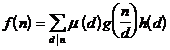The opposite is true .

4 ° Mobius transform Let n be a positive integer , if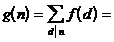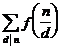but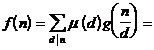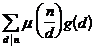g ( n ) is called the Mobius transform of f ( n ) , and f ( n ) is called the inverse Mobius transform of g ( n ) .

5 ° product Mobius transform Let n be a positive integer , if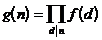but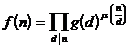g ( n ) is called the product Mobius transform of f ( n ) , and f ( n ) is called the inverse product Mobius transform of g ( n ) .

[ Mobius transformation table ]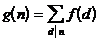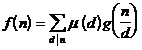g ( n ) f ( n )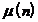d ( n )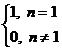1d ( n ) 1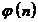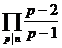n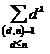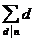n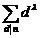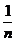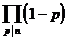L ( n ) - log nlog n L ( n )